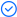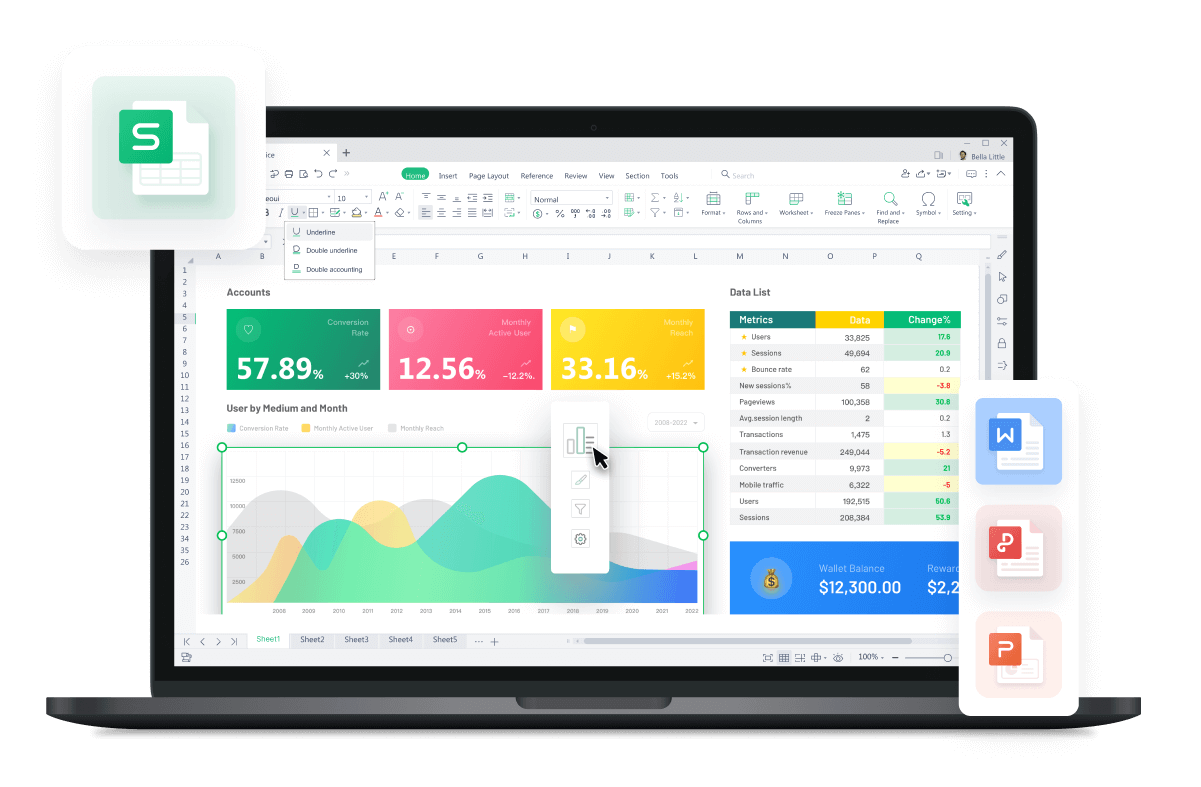WPS Office

Free All-in-One Office Suite with PDF EditorRead, edit, and convert PDFs with the powerful PDF toolkit.Microsoft-like interface, easy to use.

Windows • MacOS • Linux • iOS • Android# How to add cells in excel with formula (Easy Steps)

August 5, 2022
4.6K Views

Looking to add cells formula in Excel but not sure how? Look no further! In this article, we will show you the easiest way to add cells in Excel 2016 and 2019 using the keyboard.

If you want to add cells formula in Excel 2017, 2019 or later for the Mac, there are a few different ways to do it. One way is to use the mouse, but this can be time-consuming and awkward. Another way is to use keyboard shortcuts, but this can be difficult to learn. there is a good chance you will want to use the formula bar. However, if you are not familiar with it, this can be a bit daunting.

A Formula is an expression which calculates the value of a cell functions are predefined formulas and already available in excel.

For Example, Cell A3 below contains the SUM function which calculate the sum of the range A1:A2.

Enter a Formula Add Cells In Excel 2016/2019.

To Enter a Formula, execute the following steps.

1.Select a cell.

2.To let Excel know that you want to enter a formula, type an equal sign(=).

3.For example, type the formula A1+A2

4.Tip instead of using A1 and A2, simply select cell A1 and A2.

5.Change the value of cell A1 to 5.

Excel automatically recalculate the value of cell A3.This is one of Excel’s most powerful features!

Edit a Formula Add Cells In Excel 2016/2019 Mac

1.When you select a cell excel show the value or formula of the cell in the formula bar.

2.To edit a formula click in the formula bar and change the formula.

3.Press Enter.

Operator Precedence

Excel uses a default order in which calculations occur. If a part of the formula is in parentheses that part will be calculated first. It then performs Multiplication or division calculations. Once this is complete, excel will add and subtract the reminder of your formula see the example below.

Copy/Paste Formula Add Cells in Excel 2016/2019 Mac.

When you copy a formula excel automatically adjust the cell references for each new cell the formula is copied to, To understand this, execute the following steps

1.Enter the formula show below into cell A4.

2.Select cell A4, right click then click copy (Press ctrl+c)

3.Next select cell B4 right click then click paste under paste option(or press Ctrl + V )

4.You can also drag the formula to cell B4. Select cell A4, Click on the lower right corner of cell A4 and drag it across to cell B4. This is much easier and gives the exact same result.

5.Result the formula in cell B4 references the values in column B.

Insert Function

Every function has the same structure. For example SUM(A1:A4). The name of this function is sum. The part between the brackets (arguments) means we give excel the range A1:A4 as input. This function adds the values in cells A1,A2,A3 and A4. Its not easy to remember which function and which arguments to use for each task. Fortunately the insert function feature in excel helps you with this.

To insert a function execute the following steps

1.Select a cell.

2.Click the insert function button.

The 'insert function'dialog box appear.

1.Search for a function or select a function from a category. For example choose COUNTIF form these Statistical category.

2.Click ok.

The function argument dialog box appear

1.Click in the range box and select the range A1:C2

2.Click in the criteria box and type >5

3.Click ok.

4.Result. The COUNTIF function counts the numbers of cells that are greater than 5.

Now we come to conclusion that using a formula add cells in Excel 2016/2019 on mac can help you to add cells together quickly and easily. This can be helpful when you need to calculate a total or when you are working with a large spreadsheet. Excel is a powerful tool and learning how to use formulas can help you to become more efficient in your work. If you are not already familiar with formulas, be sure to take the time to learn how to use them.